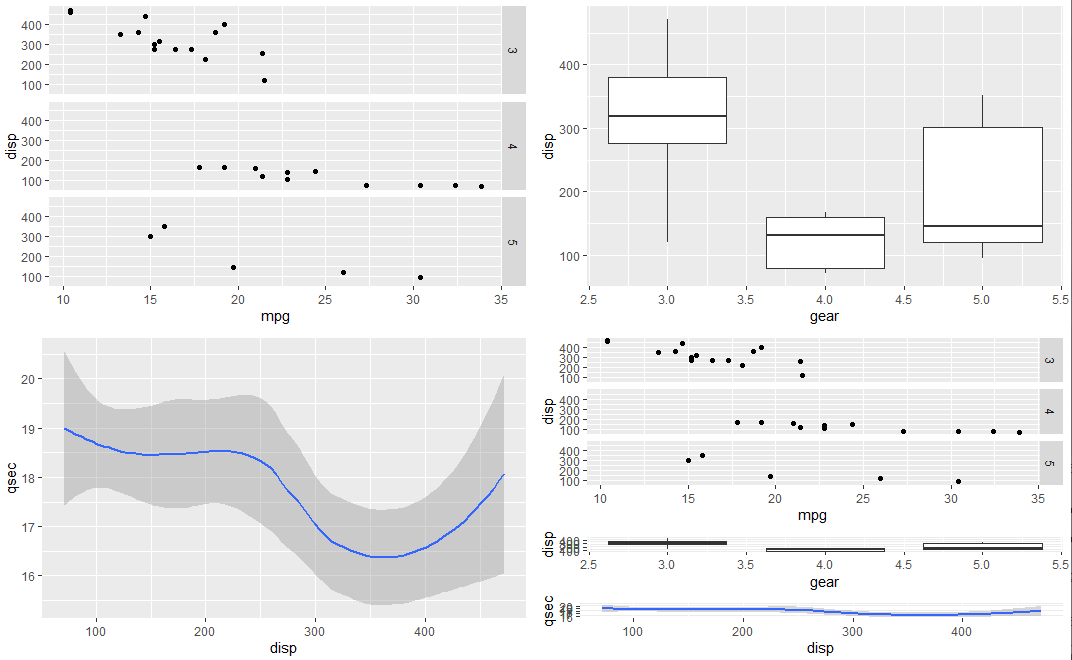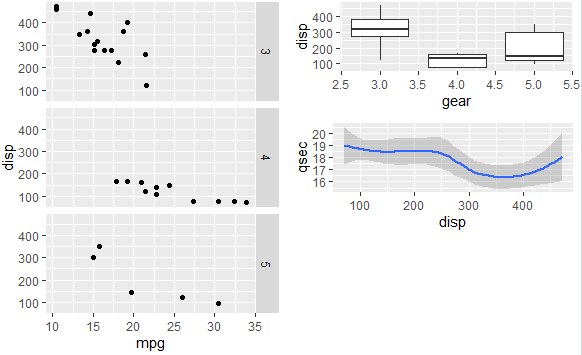3 views

With grid.arrange I can arrange multiple ggplot figures in a grid to achieve a multi-panel figure by using something like:

library(ggplot2)

library(grid)

library(gridExtra)

generate some ggplot2 plots, then

plot5 <- grid.arrange(plot4, plot1, heights=c(3/4, 1/4), ncol=1, nrow=2)

How can I obtain an 'unbalanced' 2 col layout with one plot in the entire first col and three plots in the second col? I toyed with a 'grid-of-grids' approach by trying to use grid.arrange to plot one grid (e.g.  plot5, above) against another plot, but obtained:

Error in arrangeGrob(..., as.table = as.table, clip = clip, main = main, : input must be grobs!

by

To obtain an unbalanced grid of ggplots, you can use the arrangeGrob function, since grid.arrange directly draws on the device.

For example:

library("ggplot2")

library(grid)

library(gridExtra)

data("mtcars")

p1 <- ggplot(mtcars) + geom_point(aes(mpg, disp)) + facet_grid(rows = vars(gear))

p2 <- ggplot(mtcars) + geom_boxplot(aes(gear, disp, group = gear))

p3 <- ggplot(mtcars) + geom_smooth(aes(disp, qsec))

grid.arrange(p1,p2,p3, arrangeGrob(p1,p2,p3, heights=c(3/4, 1/4, 1/4), ncol=1),

ncol=2)

Output:You can also use the layout_matrix argument as follows:

grid.arrange(p1,p2,p3, layout_matrix = cbind(c(1,1,1), c(2,3,4)))

Output: